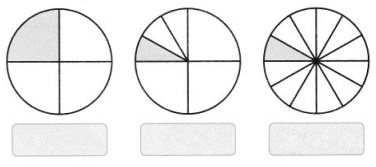# Grade 7 Fractions and Decimals Worksheets

### Grade 7 Maths Fractions and Decimals Multiple Choice Questions (MCQs)

1. Which of the following fractions has denominator 8?
(a)$\frac{8}{3}$
(b) 1$\frac{3}{5}$
(c) 1$\frac{8}{3}$
(d)$\frac{3}{8}$

2. Which one of the followings is a proper fraction?
(a)$\frac{7}{5}$
(b)$\frac{3}{2}$
(c)$\frac{4}{7}$
(d)$\frac{4}{3}$

3. Which one of the followings is an improper fraction?
(a)$\frac{2}{3}$
(b)$\frac{5}{7}$
(c)$\frac{7}{4}$
(d)$\frac{1}{2}$

4. What is the value of$\frac{2}{3}+\frac{1}{3}+\frac{7}{3}$?
(a)$\frac{10}{3}$
(b)$\frac{10}{9}$
(c)$\frac{30}{3}$
(d)$\frac{10}{27}$

5. What is the value of$\frac{4}{5}-\frac{2}{3}$?
(a)$\frac{2}{2}$
(b)$\frac{14}{15}$
(c)$\frac{2}{15}$
(d) none of these

6. The value of$\frac{1}{2}$ of 24 is:
(a) 12
(b)$\frac{1}{12}$
(c) 48
(d)$\frac{1}{48}$

7. Which of the following product gives the value$\frac{78}{5}$?
(a) 3 × 5$\frac{1}{5}$
(b)$\frac{1}{3}$
(c) 3 ×$\frac{5}{26}$
(d) none of these

8. The reciprocal of 1$\frac{2}{3}$ is:
(a)$\frac{3}{2}$
(b) 1$\frac{3}{2}$
(c)$\frac{5}{3}$
(d)$\frac{3}{5}$

9. The value of 3$\frac{1}{2}$ of$\frac{8}{3}$ is:
(a) 4
(b)$\frac{28}{3}$
(c)$\frac{9}{4}$
(d)$\frac{21}{16}$

10. The value of 4$\frac{1}{3}$ of 3 is:
(a) 4
(b) 13
(c)$\frac{13}{9}$
(d)$\frac{9}{13}$

11. Which of the following is the least form of$\frac{18}{36}$?
(a)$\frac{3}{6}$
(b)$\frac{9}{18}$
(c)$\frac{1}{2}$
(d)$\frac{2}{1}$

12. What is the sum of 5.300 and 3.250?
(a) 8.550
(b) 85.50
(c) 5.6250
(d) 8550

13. What is the value of 29.35 – 04.56?
(a) 23.75
(b) 16.35
(c) 16.25
(d) 24.79

14. Which one of the following is greater?
(a) 5.0
(b) 0.5
(c) 0.005
(d) 0.05

15. Which one of the following is smaller?
(a) 2.031
(b) 2.301
(c) 0.2301
(d) 23.01

16. 5 cm in Km can be written as:
(a) 0.0005
(b) 0.00005
(c) 0.005
(d) 0.05

17. The place value of 2 in 21.38 is:
(a) Ones
(b) Tens
(c) Tenth
(d) Hundredth

18. The value of 1.3 × 3.1 is:
(a) 403
(b) 0.403
(c) 4.03
(d) 0.0403

19. The value of 0.80 ÷ 5 is:
(a) 16
(b) 0.16
(c)$\frac{1}{16}$
(d) 1.6

20. The value of 7.75 ÷ 0.25 is:
(a) 31
(b) 0.0031
(c) 0.31
(d) 3.1

### Grade 7 Maths Fractions and Decimals Fill In The Blanks

1.$\frac{1}{2}+\frac{2}{3}=$$\square$
2.$\frac{5}{7}-\frac{2}{3}=$$\square$
3.$\frac{2}{3} \times \frac{5}{7}=$$\square$
4.$\frac{2}{3} \times \frac{9}{20}=$$\square$
5. The two-third of 42 is ………….. .
6. 3$\frac{3}{4}$ +$\square$ = 4
7. 12 – 2$\frac{3}{4}$ =$\frac{\square}{4}$
8. 0.01 × 0.01 =$\square$
9. 3$\frac{1}{5}$ ÷ 1$\frac{2}{3}$ =$\square$
10. 7.75 ÷ 0.25 =$\square$

### Grade 7 Maths Fractions and Decimals Very Short Answer Type Questions

1. Divide$\frac{15}{24}$ by$\frac{3}{4}$.
2. How long is 88.6 km than 48 km?
3. If cost of 20 pens is ₹ 356.80, then find the cost of a pen.
4. Express in kg:
(a) 4730g
(b) 8kg 4g
5. Convert:
(a) 0.25 into fraction
(b)$\frac{19}{20}$ to decimal form
6. Each side of a square field is 5.5m. Find the perimeter of the square field.

1. Karim had 20 toffees. He took these toffees with him to a picnic with four of his friends. Karim along with friends, shared these toffees equally. How many toffees did each one get?
2. Kartikeya bought 5kg 500g apples and 3kg 250g of oranges. Shivani bought 4kg 200g mangoes and 4kg 500g guavas. Who bought more fruits and how much?
3. Soumya ate$\frac{2}{5}$ part of an apple and her younger brother Somu ate the remaining part. What part of the apple did Sonu eat? Who had the longer share and by how much?
4. Write the pictorial representation of shaded portion in the form of fraction.2. How many pieces of length 2$\frac{2}{5}$ metres can be cut from 6$\frac{1}{5}$ metres of cloth?